# Semiconductor Diode

Semiconductor diode – type of diode, which contains “p-n junction” made from differently doped semiconductor materials. It is double-ended, nonlinear electronic component, where terminal attached to the “p” layer (+) is called anode and “n” layer () cathode. This electronic component is mainly used because of it’s ability of making electric current flow only in one direction (from anode to cathode) after forward-biasing the aforementioned “p-n junction” with the positive electric voltage.

However, in the opposite direction (reverse bias of the “p-n” junction with the negative electric voltage) we can say that in ideal semiconductor diode electric current won’t flow. This is why semiconductor diode is often described as the “electric valve”, which can pass or block the flow of the electric current.

## P-N Junction

If P-type semiconductor is joined with N-type semiconductor, a P-N junction is formed. The region in which the P-type and N-type semiconductors are joined is called P-N junction. This p-n junction separates n-type semiconductor from p-type semiconductor.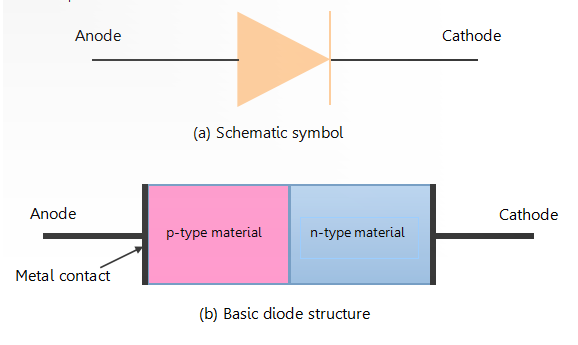## Formation of Depletion Layer

Diode is formed by joining p-type and n-type semiconductor material.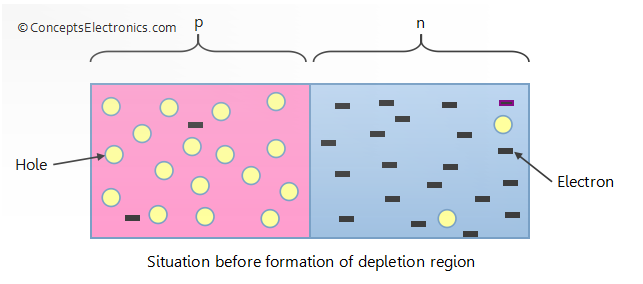• When the two types of semiconductor materials are joined together, the electrons from the n-type material diffuse into p-type material and combines with holes.
• This creates a layer of negative ions near the junction in p-type material. Negative ions are formed because the trivalent impurities ( e.g. Aluminum) now has an extra electron from the n-type material.
• Similarly the holes from the p-type material diffuse into n-type material resulting in a layer of positive ions in the n-type material.
•  These two layers of positive and negative ions form the depletion region. The term “depletion” refers to the fact that the region near the junction is depleted of their respective majority charge carriers.

The figure below shows the condition after the formation of depletion region.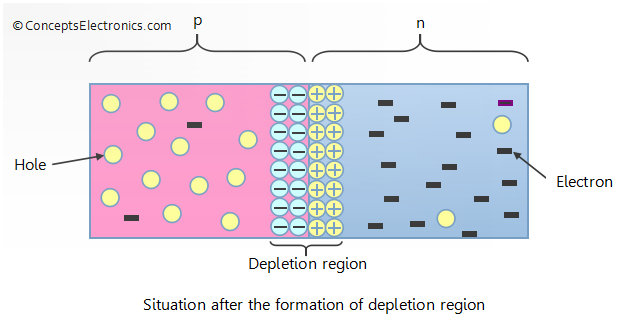The diffusion does not occur indefinitely and it stops after a quick span of time and the depletion region is said to be completely formed. Take a look at the figure below to understand how this happens.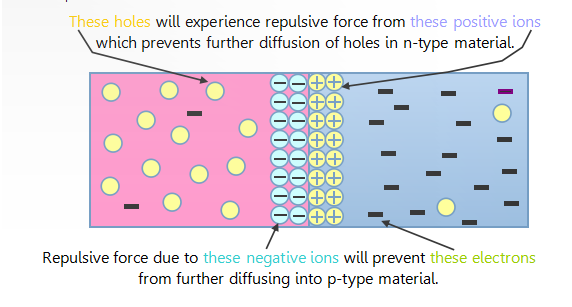## The behavior of P-N junction

(I) Forward Biased

(II) Reverse Biased

### Forward Bias

In forward bias, the P-type is connected with the positive terminal and the n-type is connected with the negative terminal. ith a battery connected this way, the holes in the P-type region and the electrons in the N-type region are pushed toward the junction. This reduces the width of the depletion zone.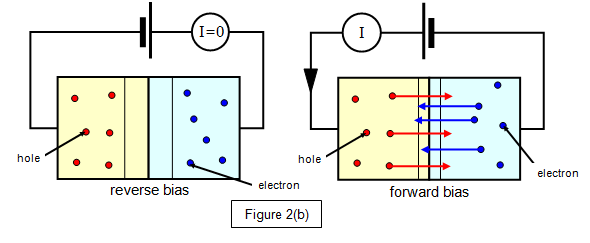### Reverse Bias

Connecting the P-type region to the negative terminal of the battery and the N-type region to the positive terminal corresponds to reverse bias. If a diode is reverse-biased, the voltage at the cathode is comparatively higher than the anode. Therefore, no current will flow until the diode breaks down. The connections are illustrated in the diagram to the right.

## Static V-I Characteristics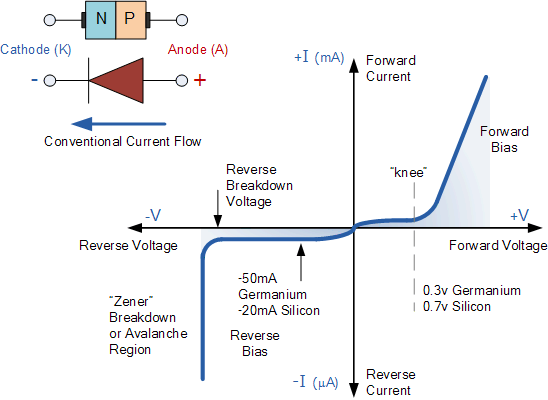## Ideal Diode

A diode is said to be an Ideal Diode when it is forward biased and acts like a perfect conductor, with zero voltage across it. Similarly, when the diode is reversed biased, it acts as a perfect insulator with zero current through it. The V-I characteristics of the Ideal diode are shown in the figure below.An Ideal diode also acts like a switch. When the diode is forward biased it acts like a closed switch as shown in the figure below.Whereas, if the diode is reversed biased, it acts like an open switch as shown in the figure below.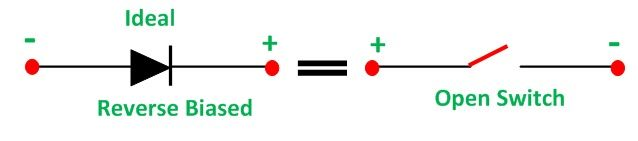## Practical Diode or Real Diode

Real diode contains barrier potential V0 (0.7 V for silicon and 0.3 V for Germanium) and a forward resistance RF of about 25 ohms. When a diode is forward biased and conducts a forward current IF flows through it which causes a voltage drop IFRF in the forward resistance. Hence, the forward voltage Vapplied across the real diode for conduction, has to overcome the following.

• Potential barrier
• Drop in forward resistance

i.e.,For Silicon diode, the equation becomes as shown below.For Germanium diode the equation becomesThe V-I characteristic of the Real diode is shown below.

##For all the practical purposes, a diode is considered to be an open switch when reversing biased. It is because the value of reverse resistance is so high (RR > 100 MΩ) that is considered to be an infinite for all practical purposes.

The equivalent circuit of the real diode under forwarding bias condition is shown below.

##This circuit shows that a real diode still acts as a switch when forward biased, but the voltage required to operate this switch is VF, that is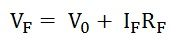References: https://semesters.in